Next: The equipartition theorem Up: Applications of statistical thermodynamics Previous: Ideal monatomic gases

What has gone wrong? First of all, let us be clear why Eq. (442) is incorrect.

We can see that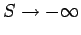as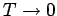, which contradicts the third law of thermodynamics. However, this is not a problem. Equation (442) was derived using classical physics, which breaks down at low temperatures. Thus, we would not expect this equation to give a sensible answer close to the absolute zero of temperature.

Equation (442) is wrong because it implies that the entropy does not behave properly as an extensive quantity. Thermodynamic quantities can be divided into two groups, extensive and intensive. Extensive quantities increase by a factor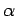when the size of the system under consideration is increased by the same factor. Intensive quantities stay the same. Energy and volume are typical extensive quantities. Pressure and temperature are typical intensive quantities. Entropy is very definitely an extensive quantity. We have shown [see Eq. (426)] that the entropies of two weakly interacting systems are additive. Thus, if we double the size of a system we expect the entropy to double as well. Suppose that we have a system of volumecontainingmoles of ideal gas at temperature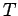. Doubling the size of the system is like joining two identical systems together to form a new system of volume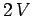containing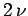moles of gas at temperature. Let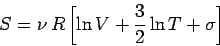(444)

denote the entropy of the original system, and let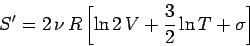(445)

denote the entropy of the double-sized system. Clearly, if entropy is an extensive quantity (which it is!) then we should have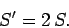(446)

But, in fact, we find that(447)

So, the entropy of the double-sized system is more than double the entropy of the original system.

Where does this extra entropy come from? Well, let us consider a little more carefully how we might go about doubling the size of our system. Suppose that we put another identical system adjacent to it, and separate the two systems by a partition. Let us now suddenly remove the partition. If entropy is a properly extensive quantity then the entropy of the overall system should be the same before and after the partition is removed. It is certainly the case that the energy (another extensive quantity) of the overall system stays the same. However, according to Eq. (447), the overall entropy of the system increases byafter the partition is removed. Suppose, now, that the second system is identical to the first system in all respects except that its molecules are in some way slightly different to the molecules in the first system, so that the two sets of molecules are distinguishable. In this case, we would certainly expect an overall increase in entropy when the partition is removed. Before the partition is removed, it separates type 1 molecules from type 2 molecules. After the partition is removed, molecules of both types become jumbled together. This is clearly an irreversible process. We cannot imagine the molecules spontaneously sorting themselves out again. The increase in entropy associated with this jumbling is called entropy of mixing, and is easily calculated. We know that the number of accessible states of an ideal gas varies with volume like. The volume accessible to type 1 molecules clearly doubles after the partition is removed, as does the volume accessible to type 2 molecules. Using the fundamental formula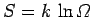, the increase in entropy due to mixing is given by(448)

It is clear that the additional entropy, which appears when we double the size of an ideal gas system by joining together two identical systems, is entropy of mixing of the molecules contained in the original systems. But, if the molecules in these two systems are indistinguishable, why should there be any entropy of mixing? Well, clearly, there is no entropy of mixing in this case. At this point, we can begin to understand what has gone wrong in our calculation. We have calculated the partition function assuming that all of the molecules in our system have the same mass and temperature, but we have never explicitly taken into account the fact that we consider the molecules to be indistinguishable. In other words, we have been treating the molecules in our ideal gas as if each carried a little license plate, or a social security number, so that we could always tell one from another. In quantum mechanics, which is what we really should be using to study microscopic phenomena, the essential indistinguishability of atoms and molecules is hard-wired into the theory at a very low level. Our problem is that we have been taking the classical approach a little too seriously. It is plainly silly to pretend that we can distinguish molecules in a statistical problem, where we do not closely follow the motions of individual particles. A paradox arises if we try to treat molecules as if they were distinguishable. This is called Gibb's paradox, after the American physicist Josiah Gibbs who first discussed it. The resolution of Gibb's paradox is quite simple: treat all molecules of the same species as if they were indistinguishable.

In our previous calculation of the ideal gas partition function, we inadvertently treated each of themolecules in the gas as distinguishable. Because of this, we overcounted the number of states of the system. Since the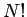possible permutations of the molecules amongst themselves do not lead to physically different situations, and, therefore, cannot be counted as separate states, the number of actual states of the system is a factorless than what we initially thought. We can easily correct our partition function by simply dividing by this factor, so that(449)

This gives(450)

or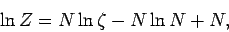(451)

using Stirling's approximation. Note that our new version ofdiffers from our previous version by an additive term involving the number of particles in the system. This explains why our calculations of the mean pressure and mean energy, which depend on partial derivatives ofwith respect to the volume and the temperature parameter, respectively, came out all right. However, our expression for the entropyis modified by this additive term. The new expression is(452)

This gives(453)

where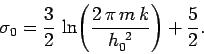(454)

It is clear that the entropy behaves properly as an extensive quantity in the above expression: i.e., it is multiplied by a factorwhen,, andare multiplied by the same factor.Next: The equipartition theorem Up: Applications of statistical thermodynamics Previous: Ideal monatomic gases
Richard Fitzpatrick 2006-02-02